CE433 Embedded Devices Spring 2022
Homework 8 - Softcore
Name: Ryan Jeanes

Email: rejeanes@fortlewis.edu

Homework 8 - Softcore
Introduction
This assignment introduced  Pico Blaze and KCPSM6 architecture.
Results
All of the tasks were succesfully completed.
Section 3

This is the demonstration video for section 3.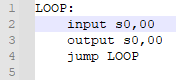Figure 1 - Section 3 .psm code
Section 4
This is the demonstration video for section 4.

Section 5
This is the demonstration video for section 5.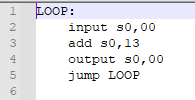Figure 2 - Code to add 0x13 to output

Section 6
This is the demonstration video for section 6.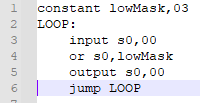Figure 3 - Code to mask low 2 bits for output

Section 7
This is the demonstration video for section 7.Figure 4 - Code to shift output 1 bit right from input
Section 8
This is the demonstration video for section 8.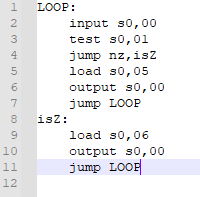Figure 5 - Code that outputs 0x06 if test,01 returns 0, and 0x05 if not zero.
Section 9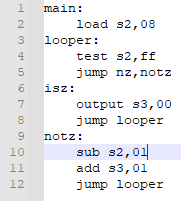Figure 6 - Code that increments output by 1 when s2 reaches 0 using test instruction.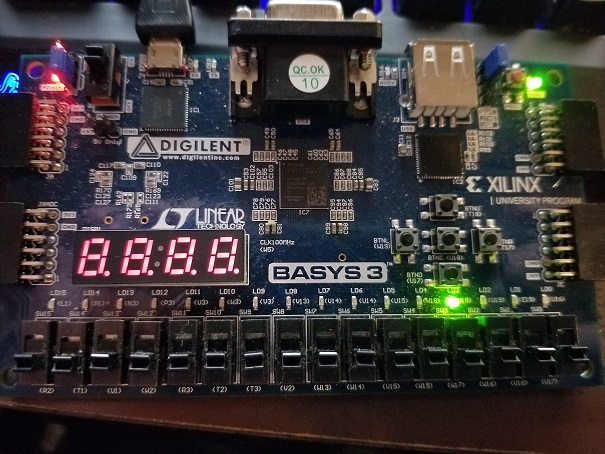Figure 7 - Picture of section 9 code being executed.
Section 10Figure 8 - Section 10 code that does the same as section 9, but using the compare instructionFigure 9 - Picture of section 10 code being executed.